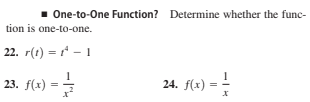# 1 One-to-One Function? Determine whether the func- tion is one-to-one. 22. r(t) = * - 1 23. (1) - 4 23. f(x) = 24. f(x) = -

Questionhelp_outlineImage Transcriptionclose1 One-to-One Function? Determine whether the func- tion is one-to-one. 22. r(t) = * - 1 23. (1) - 4 23. f(x) = 24. f(x) = - fullscreen

### Want to see this answer and more?

Experts are waiting 24/7 to provide step-by-step solutions in as fast as 30 minutes!*

*Response times may vary by subject and question complexity. Median response time is 34 minutes for paid subscribers and may be longer for promotional offers.
Tagged in
MathCalculus

### Functions